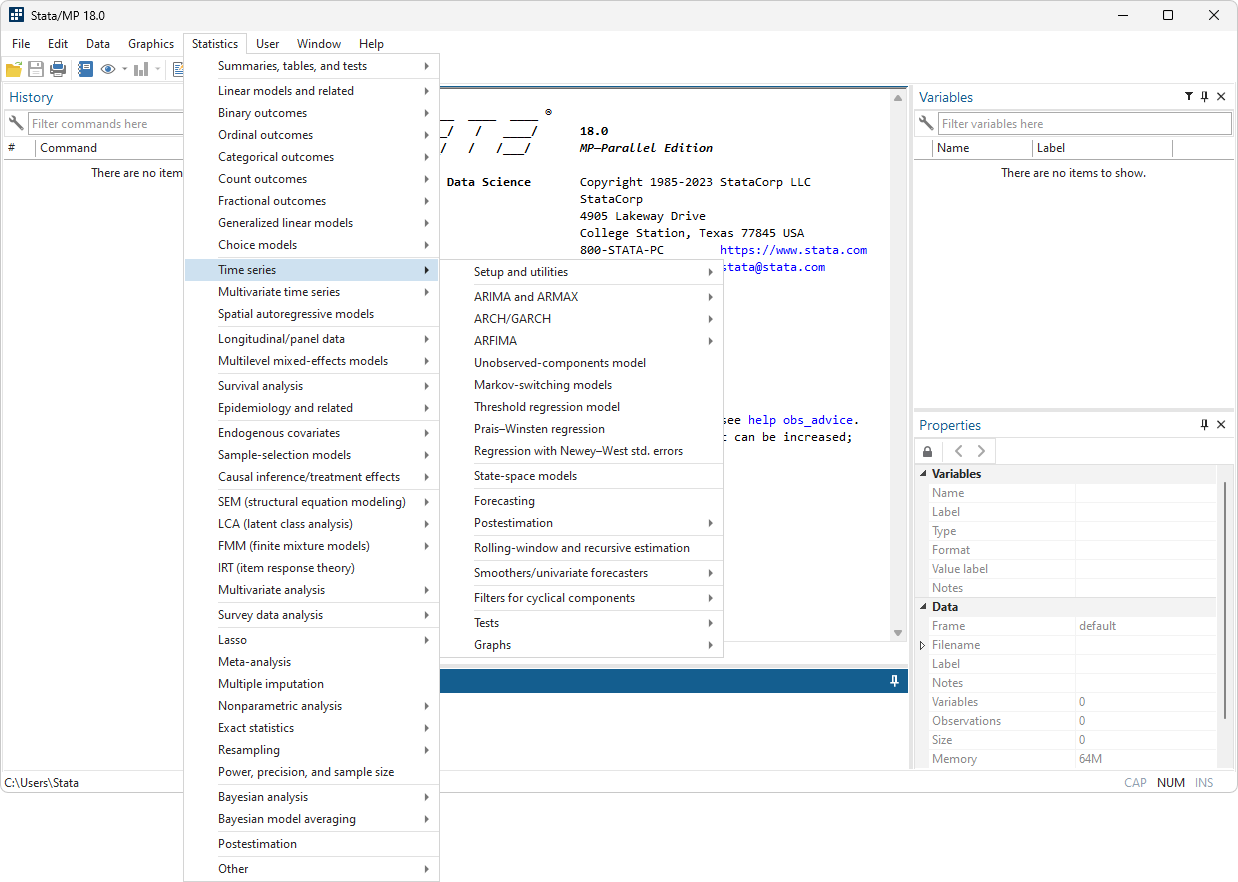Handle all the statistical challenges inherent to time-series data—autocorrelations, common factors, autoregressive conditional heteroskedasticity, unit roots, cointegration, and much more. From graphing and filtering to fitting complex multivariate models, let Stata reveal the structure in your time-series data.

ARIMA

• ARMA
• ARMAX
• Standard and robust variance estimates
• Static and dynamic forecasts
• Linear constraints
• Multiplicative seasonal ARIMA
• Spectral densities
• Impulse–response functions (IRFs)
• Parametric autocorrelation estimates and graphs
• Check stability conditions
• Model selection criteria

ARCH/GARCH

• GARCH
• APARCH
• EGARCH
• NARCH
• AARCH
• GJR and more
• ARCH in mean
• Standard and robust variance estimates
• Normal, Student’s t, or generalized error distribution
• Multiplicative deterministic heteroskedasticity
• Static and dynamic forecasts
• Linear constraints

MULTIVARIATE GARCH

• Diagonal VECH models
• Conditional correlation models
• Constant conditional correlation
• Dynamic conditional correlation
• Varying conditional correlation
• Multivariate normal or multivariate Student’s t errors
• Standard and robust variance estimates
• Static and dynamic forecasts
• Linear constraints

MARKOV-SWITCHING MODELS

• Dynamic regression
• Autoregression
• Tables of transition probabilities
• Tables of expected durations
• Standard and robust variance estimates

ARFIMA

• Long-memory processes
• Fractional integration
• Standard and robust variance estimates
• Static and dynamic forecasts
• Linear constraints
• Spectral densities
• Impulse–response functions (IRFs)
• Parametric autocorrelation estimates and graphs
• Model selection criteria

REGRESSION WITH AR(1) DISTURBANCES

• Heteroskedasticity-and-autocorrelation-consistent covariance matrices
• Cochrane–Orcutt/Prais–Winsten methods
• ARMA/ARIMA estimators
• ARCH estimators

UNOBSERVED COMPONENTS MODEL (UCM)

• Trend-cycle decomposition
• Stochastic cycles
• Estimation by state-space methods
• Standard and robust variance estimates
• Static and dynamic forecasts
• Linear constraints
• Spectral densities

FRED DATA

• Over 566,000 U.S. and international economic and financial time series
• Search or browse by subject, title, or source
• Put series on a common periodicity
• Easily update datasets containing dozens, or even hundreds, of series
• Easy-to-use interface for searching and browsing
• Commands for updating datasets and replicability

• Create calendar from dataset
• Format variables using business calendar format
• Convert between business dates and regular dates
• Lags and leads calculated according to calendar

GRAPHS AND TABLES

• Autocorrelations and partial correlations
• Cross-correlations
• Cumulative sample spectral density
• Periodograms
• Line plots
• Range plot with lines
• Patterns of missing data

TIME-SERIES FUNCTIONS

• String conversion to date: daily, weekly, monthly, quarterly, half-yearly, yearly
• Dates and times from numeric arguments
• Date and time literal support
• Periodicity conversion, e.g., daily date to quarterly
• Date and time ranges

TIME-SERIES OPERATORS

• L, lag
• D, differences
• S#, seasonal lag

TIME-SERIES TIME AND DATE FORMATS

• Default formats for clock-time daily, weekly, monthly, quarterly, half-yearly, yearly
• High-frequency data with millisecond resolution
• User-specified formats

TIME-SERIES FILTERS

• Baxter–King band-pass filter
• Butterworth high-pass filter
• Christiano–Fitzgerald band-pass filter
• Hodrick–Prescott high-pass filter

TIME-SERIES SMOOTHERS

• Moving average (MA)
• Single exponential
• Double exponential

• Holt–Winters nonseasonal exponential
• Holt–Winters seasonal exponential
• Nonlinear
• Forecasting and smoothing

SUPPORT FOR HAVER ANALYTICS DATABASE

• Import haver command makes using Haver datasets even easier
• Quickly access worldwide economics and financial datasetsVAR/SVAR/VECM

• Vector autoregression (VAR)
• Structural vector autoregression (SVAR)
• Vector error-correction models (VECM)
• Impulse–response functions (IRFs)
• Simple IRFs
• Orthogonalized IRFs
• Structural IRFs
• Cumulative IRFs
• Dynamic multipliers
• Forecast-error variance decompositions (FEVD)
• Static and dynamic forecasts
• Diagnostics and tests
• Cointegration tests
• Granger causality tests
• LM tests for residual autocorrelation
• Tests for normality of residuals
• Lag-order selection statistics
• Stability analysis using eigenvalues
• Wald lag-exclusion statistics
• Graphical and tabular presentations and comparisons of IRFs and FEVDs
• Bayesian VAR
• Lagged endogenous and exogenous variables
• Minnesota priors, including conjugate and original
• Multiple chains
• Control MCMC sampling
• Check parameter stability
• Dynamic forecasts
• IRF and FEVD analysis
• Standard Bayesian postestimation
Read about the DSGE features, including the new Bayesian estimation for linear and nonlinear DSGEs.

LOCAL PROJECTIONS

• Local projection estimation
• Impulse–response functions (IRFs)
• Simple IRFs
• Orthogonalized IRFs
• Dynamic multipliers
• Graphical and tabular presentations and comparisons of IRFs

FORECAST MODELS

• Combine results from multiple estimation commands
• Specify identities and declare exogenous variables
• Obtain dynamic and static forecasts
• Use simulation methods to obtain prediction intervals
• Specify alternative scenarios and perform “what-if” analyses
• Bayesian dynamic forecast after VAR

STATE-SPACE MODELS

• VARMA models
• Structural time-series models
• Stochastic general-equilibrium models
• Stationary and nonstationary models
• Standard and robust variance estimates
• Static and dynamic forecasts
• Linear constraints

DYNAMIC-FACTOR MODELS

• Unobserved factors with vector autoregressive structure
• Exogenous covariates
• Autocorrelated disturbances in dependent variables’ equations
• Standard and robust variance estimates
• Static and dynamic forecasts
• Linear constraints

THRESHOLD REGRESSION

• One threshold or multiple thresholds
• Specify number of thresholds
• Automatically choose the number of thresholds, using
• BIC
• AIC
• Hannan-Quinn information criterion
• Thresholds may be:
• Points in time
• Values of covariates in the regression model
• Values of variables not in the regression model
• Bayesian threshold autoregressive models

TESTS FOR STRUCTURAL BREAKS

• Unknown break point
• Known break points
• Cumulative sum test for stability of coefficients

POSTESTIMATION SELECTOR

• View and run all postestimation features for your command
• Automatically updated as estimation commands are run

TESTS FOR WHITE NOISE

• Portmanteau’s test
• Bartlett’s periodogram test

REGRESSION DIAGNOSTICS

• LM test for ARCH effects
• Breusch–Godfrey LM test for serial correlation
• Durbin alternative test for serial correlation
• Durbin–Watson statistic

TESTS FOR UNIT ROOTS

• Dickey–Fuller
• Modified Dickey–Fuller t test proposed by Elliott, Rothenberg, and Stock
• Augmented Dickey–Fuller test
• Phillips–Perron

ROLLING AND RECURSIVE ESTIMATION